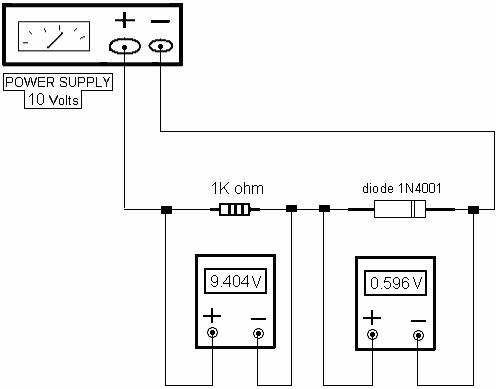SILICON DIODE: The schematic symbol of a silicon diode and its equivalent

model (a switch connected to a 0.7 V battery) is shown in figure 1.3.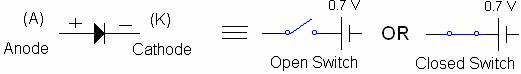Figure 1.3

Note the following:

VA is the anode voltage of the diode.

VK is the cathode voltage of the diode.

VD = VAVK.

A silicon diode works based on the following:

If VD > 0.7, the switch is CLOSED in figure 1.3 (diode is conducting or forward biased). If VD < 0.7, the switch is OPEN in figure 1.3 (diode is not conducting or reverse biased). Figure 1.4 demonstrates the functions of the diode.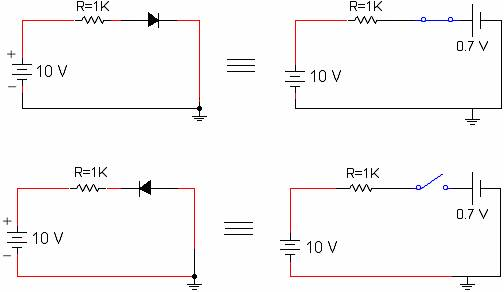Figure 1.4

Note that when the diode is conducting, VR = 9.3 volts (10 - 0.7) and when the diode is not conducting, VR = 0 volts. The purpose of resistor R is to limit the current in the circuit so

the diode would not be damaged.

The diode electrical characteristics is available in the Specification (spec) Sheet which lists all the parameters such as current rating, power rating, peak reverse voltage rating, and etc. Figure 1.5 shows a silicon diode with its schematic symbol.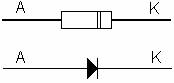Figure 1.5

Lab 1.2    Part One

Set up the circuit (software).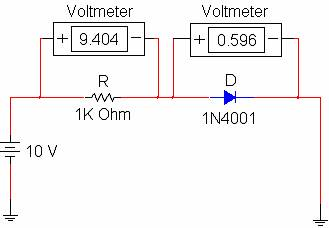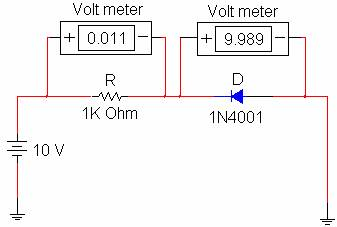1. In the first circuit, diode is forward biased (conducting or CLOSED switch). The voltage across the diode is about 0.6 volts and the voltage across the resistor is

9.4 volts.

1. In the second circuit, diode is reverse biased (non-conducting or OPEN switch). Since the current is about zero in the circuit, the voltage across the resistor is about zero. Therefore, all the source voltage (about 10 V) is applied across the

non-conducting diode.

Lab 1.2    Part Two

Set up the circuit diagram on page 5 using the following parts: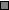10 Volts Power supplyOne diode 1N4001One 1K Ohm Resistor (1/4 W)Wire, 22 GaugeTwo Voltmeters